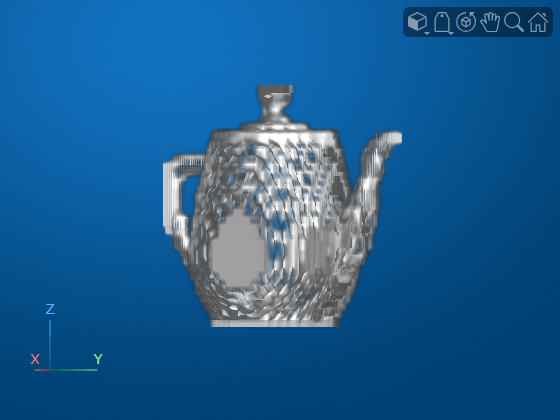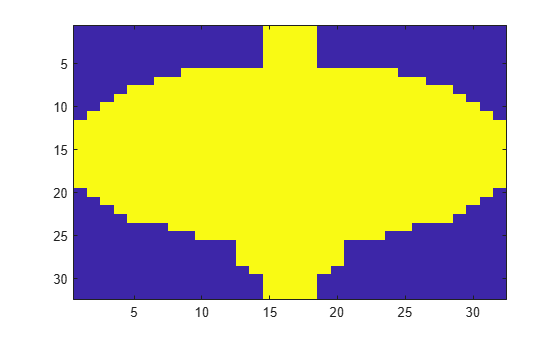pcbin

Spatially bin point cloud points

Description

example

bins = pcbin(ptCloud,numBins) spatially grids points in the point cloud, ptCloud. The output bins stores spatial bin locations, which are the indices for the points. The function finds the bin locations by sampling the point cloud in each dimension by the number of bins specified by numBins.

bins = pcbin(ptCloud,numBins,spatialLimits) specifies the spatial limits to use when the function places points into bins.

[bins,binLocations] = pcbin(___) also returns binLocations, the spatial bounds of each bin in bins. binLocations is the same size as bins.

[___] = pcbin(___,'BinOutput',true) also specifies the format of the bins and binLocations output.

When you set 'BinOutput' to true, bins and binLocations are returned as cell arrays of size [numBinsX,numBinsY,numBinsZ].

When you set 'BinOutput' to false and ptCloud are unorganized point clouds, bins is returned as an M-by-1 numeric vector. binLocations is returned as an M-by-6 matrix.

When you set 'BinOutput' to false and ptCloud are organized point clouds, bins is returned an M-by-N matrix. binLocations is returned as an M-by-N-by-6 matrix.

Examples

collapse all

Read point cloud data into the workspace.

Spatially bin the point cloud into a 32-by-32-by-32 grid.

indices = pcbin(ptCloud,[32 32 32]);

Build an occupany grid.

occupancyGrid = cellfun(@(c) ~isempty(c), indices);

Display the occupany grid.

ViewPnl = uipanel(figure);
volshow(occupancyGrid,'Parent',ViewPnl);Read point cloud data into the workspace.

Spatially bin the point cloud into a 32-by-32-by-1 grid.

indices = pcbin(ptCloud,[32 32 1]);

Build a density grid.

densityGrid = cellfun(@(c) ~isempty(c),indices);

Display the density grid.

figure;
imagesc(densityGrid);Input Arguments

collapse all

Point cloud, specified as a pointCloud object.

Number of bins, specified as a three-element vector of the form [numBinsX,numBinsY, numBinsZ]. The vector elements indicate the number of bins to use in each dimension, respectively.

Spatial limits, specified as a 3-by-2 numeric matrix of the form [Xmin, Xmax; Ymin, Ymax; Zmin, Zmax]. The spatial limits define the bounds used when the function bins points. Points that are outside of the specified limits are not included in the output.

Output Arguments

collapse all

Spatial bin locations, returned as a cell array of the same size as input numBins, an M-by-1 numeric vector, or an M-by-N matrix. The function returns a cell array when you do not use the 'BinOutput' argument. Each cell in the array contains indices to locations of points in the input point cloud that define the bins. Each bin includes the left bin edge, except for the last bin which includes both bin edges.

The table describes the size of bins based how you specify the 'BinOutput' argument, and whether ptCloud is unorganized or organized.

'BinOutput'bins
truecell arrays of size [numBinsX,numBinsY,numBinsZ]
false

Unorganized point clouds

M-by-1 numeric vector

, where M represents the number of points.

Organized point clouds

M-by-N matrix, where M and N represents the total number of points set up as an organized point cloud.

The function processes invalid points differently, depending on how you specify the 'BinOutput' argument. Invalid points are points with coordinates that contain Inf or NaN . When you set 'BinOutput' to true, the function ignores invalid points and does not include them in the output. When you set 'BinOutput' to false, the function returns NaN values for invalid points.

Bin spatial bounds, returned as a cell array the same size as the output bins, cell arrays of size [numBinsX,numBinsY,numBinsZ], an M-by-6 matrix, or an M-by-N-by-6 matrix . The function returns a cell array when you do not use the 'BinOutput' argument. Each cell in the array contains the spatial bounds of the bins in bins. The bounds for a bin are of the form [Xmin, Xmax; Ymin, Ymax; Zmin, Zmax].

The table describes the size of binLocations based how you specify the 'BinOutput' argument, and whether ptCloud is unorganized or organized.

'BinOutput'binLocations
truecell arrays of size [numBinsX,numBinsY,numBinsZ]
false

Unorganized point clouds:

M-by-6 matrix, where M represents the number of points located in the bin specified by the [Xmin, Xmax; Ymin, Ymax; Zmin, Zmax] spatial bound.

Organized point clouds:

M-by-N-by-6 matrix, where M and N represents the number of points in an organized point cloud, with each point located in the bin specified by the [Xmin, Xmax; Ymin, Ymax; Zmin, Zmax] spatial bound.